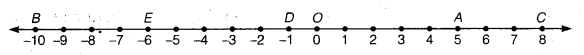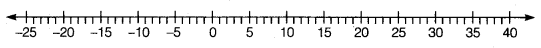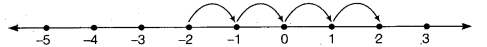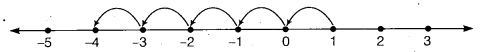# Class 6 Maths NCERT Solutions for Chapter 6 Integers Ex – 6.1

## Integers

Question 1.
Write opposites of the following:

(a) Increase in weight
(b) 30 km north
(c) 326 BC
(d) Loss of ₹ 700
(e) 100 m above sea level

Solution:
(a) Decrease in weight
(b) 30 km South
(d) Gain of ₹ 700
(e) 100 m below sea level

Question 2.
Represent the following numbers as integers with appropriate signs.

(a) An aeroplane is flying at a height two thousand metre above the ground.
(b) A submarine is moving at a depth, eight hundred metre below the sea level.
(c) A deposit of rupees two hundred.
(d) Withdrawal of rupees seven hundred.

Solution:
(a) +2000
(b) -800
(c) +200
(d) -700.

Question 3.
Represent the following numbers on a number line:

(a) +5
(b) -10
(c) +8
(d) -1
(e) -6

Solution:
In order to represent integers on a number line, we draw a line and mark a point O almost in the middle of it as shown. Now, set off equal distances on the right hand side as well as on the left hand side of O. On the right side of O, label the points of subdivisions as 1,2, 3 etc. and the point O as 0. Since negative integers are opposite of positive integers (i.e., natural numbers), so we represent negative integers in the opposite direction Le., on the left side of O on the number line. On the left side of point O, label the points of subdivision by -1, -2, -3 etc. as shown in figure.Clearly (a) +5 (b) -10 (c) +8 (d) -1 and (e) -6 are represented by the points A, B, C, D and E respectively on the number line.

Question 4.
Adjacent figure is a vertical number line, representing integers. Observe it and locate the following points:

(a) If point D is+ 8, then which point is -8?
(b) Is point G a negative integer or a positive integer?
(c) Write integers for points B and E.
(d) Which point marked on this number line has the least value?
(e) Arrange all the points in decreasing order of value.

Solution:
From the given vertical number line, clearly
(a) The point F represents -8.
(b) Since G lies below O, so it is a negative integer.
(c) Point B represents +4 and E represents -10.
(d) E has the least value.
(e) Points in decreasing order are as under: D, C, B, A, O, H, G, F, E

Question 5.
Following is the list of temperatures of five places in India, on a particular day of the year.

(a) Write the temperatures of these places in the form of integers in the blank column.
(b) Following is the number line representing the temperature in degree Celsius.Plot the name of the city against its temperature.
(c) Which is the coolest place?
(d) Write the names of the places where temperatures are above 10°C.

Solution:
(a) Siachin: -10 °C Shimla: -8°C
Srinagar: -2°C
(b) Plotting the name of the city against its temperature on the number line representing the temperature in degree Celsius, we have
(c) The coolest place is Siachin.
(d) The places having temperatures, above 10°C are Ahmedabad anti Delhi.

Question 6.
In each of the following pairs, which number is to the right of the other on the number line?

(a) 2, 9
(b) -3, -8
(c) 0, -1
(d) -11, 10
(e) -6, 6
(f) 1, -100

Solution:
(a)
9 is on the right of 2 on the number line.
(b) -3 is on the right of -8 on the number line.
(c) 0 is on the right of -1 on the number line.
(d) 10 is on the right of -11 on the number line.
(e) 6 is on the right of-6 on the number line.
(f) 1 is on the right of -100 on the number line.

Question 7.
Write all the integers between the given pairs (write them in the increasing order).

(a) 0 and -7
(b) -4 and 4
(c) -8 and -15
(d) -30 and -23

Solution:
(a)
All the integers between 0 and -7 in the increasing order are -6, -5, -4, -3, -2,-1.
(b) All the integers between -4 and 4 in the increasing order are -3, -2, -1, 0, 1, 2, 3.
(c) All the integers between -8 and -15 in the increasing order are -14, -13,-12,-11,-10,-9.
(d) All the integers between -30 and -23 in the increasing order are -29, -28, -27, -26, -25, -24.

Question 8.
(a) Write four negative integers greater than 20.
(b) Write four negative integers less than 10.

Solution:
(a)
Four negative integers greater than -20 are -20 +1, -20 + 2, -20 + 3 and -20 + 4
i. e., -19 , -18, -17 and -16.
(b) Four negative integers less than-10 are -10 -1, -10 -2, -10 -3 and -10 -4
i. e., -11, -12, -13, -14.

Question 9.
For the following statements, write true (T) or false (F). If the statement is false, correct the statement.

(a) -8 is to the right of -10 on a number line.
(b) -100 is to the right of -50 on a number line.
(c) Smallest negative integer is -1.
(d) -26 is larger than -25.

Solution:
(a)
True.
(b) False. The correct statement should be “ -100 is to the left of -50 on a number line”.
(c) False. The correct statement should be “ There is no last or smallest negative number”.
(d) False. The correct statement should be “ -26 is smaller than -25’.

Question 10.
Draw a number line and answer the following:
(a)
Which number will we reach if we move 4 numbers to the right of -2?
(b) Which number will we reach if we move 5 numbers to the left of 1?
(c) If we are at -8 on the number line, in which direction should we move to reach -13?
(d) If we are at -6 on the number line, in which direction should we move to reach -1?

Solution:
(a)
We want to obtain integer on moving 4 numbers to the right of -2. So, we start from -2 and proceed 4 units to the right of -2 to obtain 2 as shown in the figure.Hence, we will reach the number 2.
(b) We want to obtain integer on moving 5 numbers to the left of 1. So, we start from 1 and proceed 5 units to the left of 1 to obtain -4 as shown in the figure.Hence, we will reach the number -4. ,
(c) Since -13 < -8. So, to reach -13 from -8 on the number line we should move to the left of -8.
(d) Since -1 > -6. So,, to reach -1 from -6 on the number line, we should move to the right of -6.